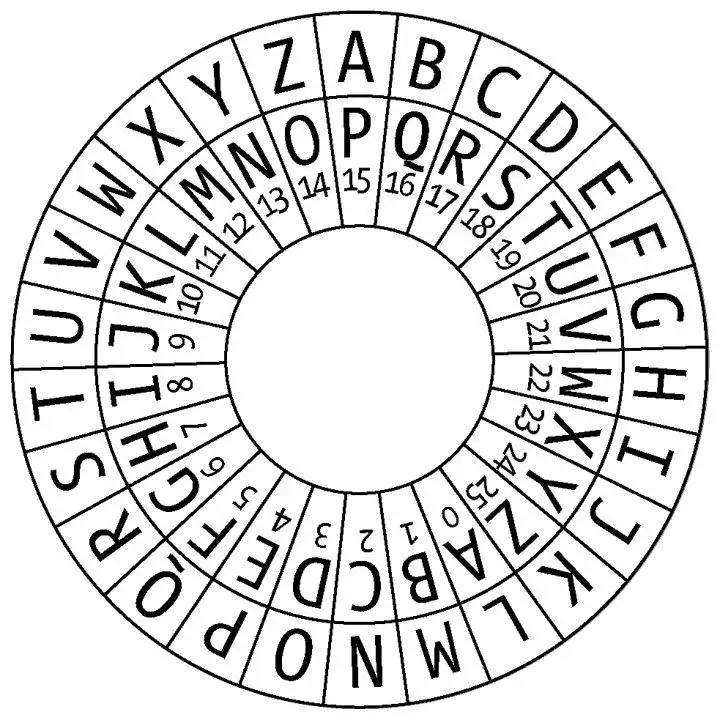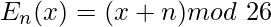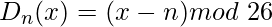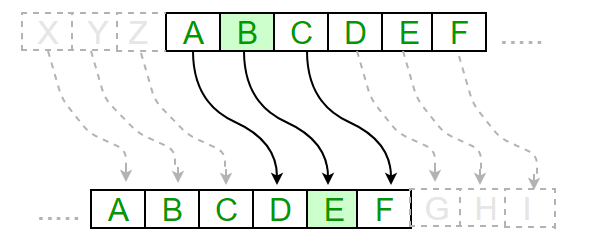（移位 n 的加密阶段）（带移位 n 的解密阶段）C++

``// A C++ program to illustrate Caesar Cipher Technique``

# include <iostream>

using namespace std;

// This function receives text and shift and
// returns the encrypted text
string encrypt(string text, int s)
{

``````string result = "";

// traverse text
for (int i=0;i<text.length();i++)
{
// apply transformation to each character
// Encrypt Uppercase letters
if (isupper(text[i]))
result += char(int(text[i]+s-65)%26 +65);

// Encrypt Lowercase letters
else
result += char(int(text[i]+s-97)%26 +97);
}

// Return the resulting string
return result;``````

}

// Driver program to test the above function
int main()
{

``````string text="ATTACKATONCE";
int s = 4;
cout << "Text : " << text;
cout << "\nShift: " << s;
cout << "\nCipher: " << encrypt(text, s);
return 0;``````

}

Python3

``#A python program to illustrate Caesar Cipher Technique``

def encrypt(text,s):

``````result = ""

# traverse text
for i in range(len(text)):
char = text[i]

# Encrypt uppercase characters
if (char.isupper()):
result += chr((ord(char) + s-65) % 26 + 65)

# Encrypt lowercase characters
else:
result += chr((ord(char) + s - 97) % 26 + 97)

return result
``````

# check the above function

text = "ATTACKATONCE"
s = 4
print ("Text : " + text)
print ("Shift : " + str(s))
print ("Cipher: " + encrypt(text,s))